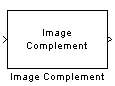# Image Complement

Compute complement of pixel values in binary or intensity images

## Library

Conversions

`visionconversions`

•## Description

The Image Complement block computes the complement of a binary or intensity image. For binary images, the block replaces pixel values equal to `0` with `1` and pixel values equal to `1` with `0`. For an intensity image, the block subtracts each pixel value from the maximum value that can be represented by the input data type and outputs the difference.

For example, suppose the input pixel values are given by x(i) and the output pixel values are given by y(i). If the data type of the input is double or single precision floating-point, the block outputs y(i) = 1.0-x(i). If the input is an 8-bit unsigned integer, the block outputs y(i) = 255-x(i).

PortInput/OutputSupported Data TypesComplex Values Supported

Input

Vector or matrix of intensity values

• Double-precision floating point

• Single-precision floating point

• Boolean

• 8-, 16-, 32-bit signed integer

• 8-, 16-, 32-bit unsigned integer

No

Output

Complement of a binary or intensity

Same as Input port

No

The dimensions, data type, complexity, and frame status of the input and output signals are the same.

 Autothreshold Computer Vision Toolbox™ software Chroma Resampling Computer Vision Toolbox software Color Space Conversion Computer Vision Toolbox software

## Extended Capabilities

### C/C++ Code GenerationGenerate C and C++ code using Simulink® Coder™.

Introduced before R2006a